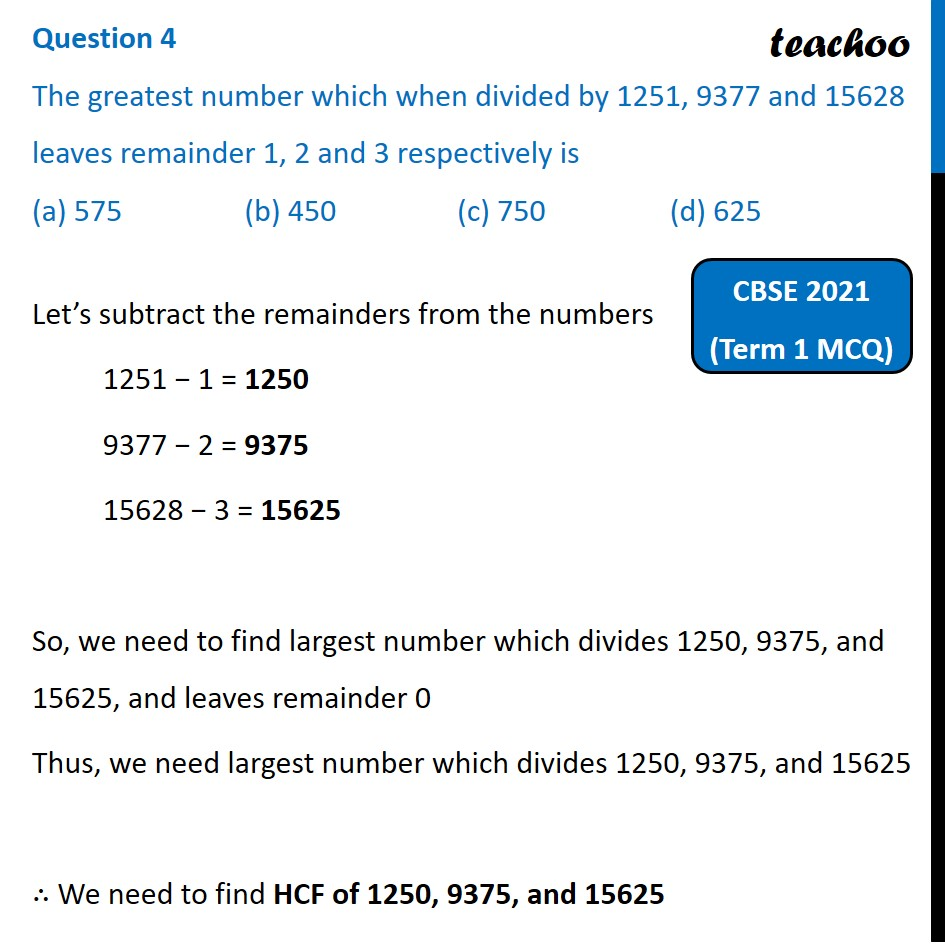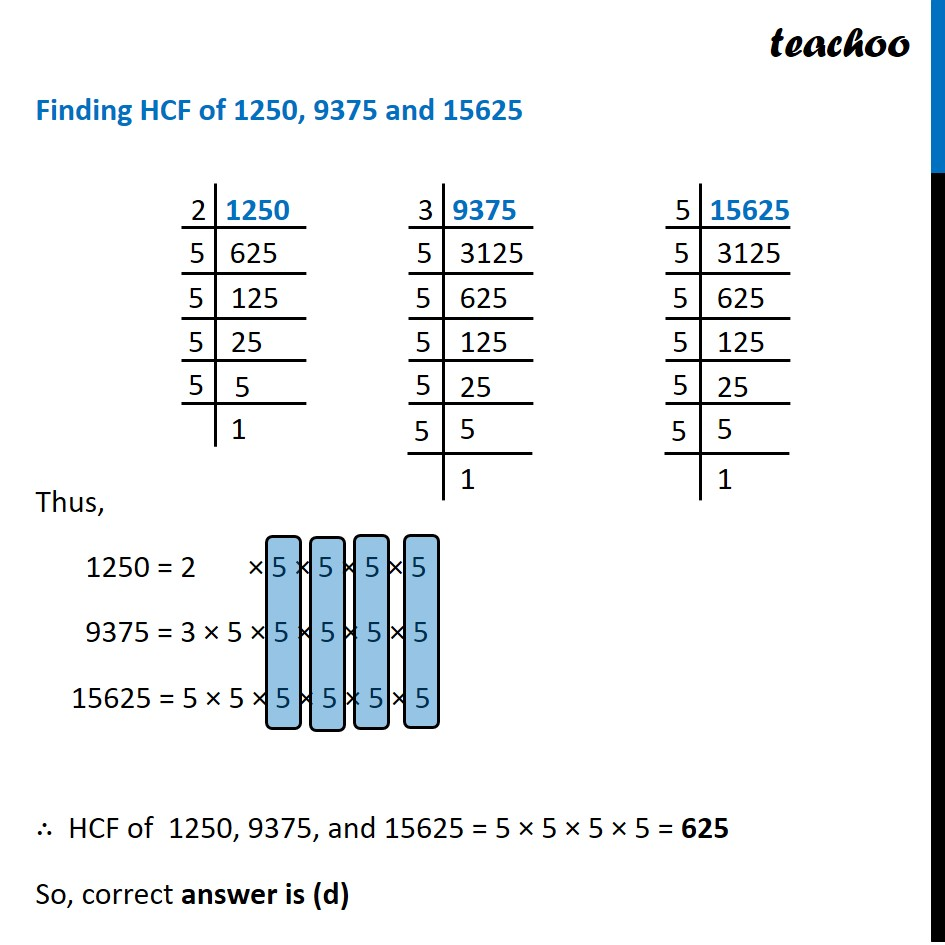Past Year MCQ (Maths Standard)

Chapter 1 Class 10 Real Numbers
Serial order wiseLearn in your speed, with individual attention - Teachoo Maths 1-on-1 Class

### Transcript

Question 4 The greatest number which when divided by 1251, 9377 and 15628 leaves remainder 1, 2 and 3 respectively is (a) 575 (b) 450 (c) 750 (d) 625Let’s subtract the remainders from the numbers 1251 − 1 = 1250 9377 − 2 = 9375 15628 − 3 = 15625 So, we need to find largest number which divides 1250, 9375, and 15625, and leaves remainder 0 Thus, we need largest number which divides 1250, 9375, and 15625 ∴ We need to find HCF of 1250, 9375, and 15625 Finding HCF of 1250, 9375 and 15625 Thus, 1250 = 2 × 5 × 5 × 5 × 5 9375 = 3 × 5 × 5 × 5 × 5 × 5 15625 = 5 × 5 × 5 × 5 × 5 × 5 ∴ HCF of 1250, 9375, and 15625 = 5 × 5 × 5 × 5 = 625 So, correct answer is (d) 2 1250 5 625 5 125 5 25 3 9375 5 3125 5 625 5 125 5 25 5 15625 15625 3125 5 625 5 125